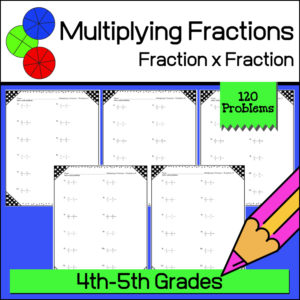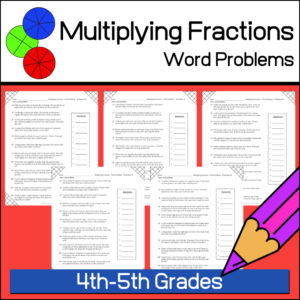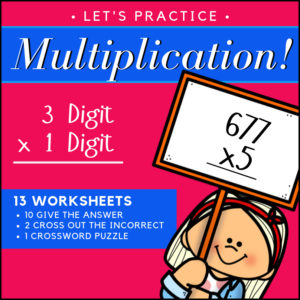Showing 1–20 of 58 results

•\$3.50

Give students the practice they need when learning to solve one-step, one-variable equations! This resource provides 7 different worksheets, each with 25 problems for a total of 175 problems. All equations in this set of worksheets use only the properties of addition and subtraction.

•\$8.00

This 38 page math glossary has been designed for 3rd-5th grades and includes 129 math terms with definitions and 72 illustrations! Terms include those surrounding basic operations, geometry and elementary statistics.

(See description below for a complete list of included terms)

•\$1.50

Help students learn and remember the difference between regular and irregular shapes by displaying this classroom poster!

•\$3.00

In geometry, students need to be able to identify a variety of irregular shapes! Here are 10 worksheets for students to practice identifying the following shapes:

• – pentagon
• – hexagon
• – nonagon
• – heptagon
• – octagon
• – decagon

•\$3.00

This resource is designed to give students the practice the need to become proficient at multiplying decimals. There are three levels, 15 worksheets (16 problems each) and answer keys. That’s 240 problems – EACH WORKSHEET includes a different growth mindset quote!
(See description below for more details)

•\$3.00

This resource is designed to give students the practice the need to become proficient at multiplying decimal numbers. There are three levels, 15 worksheets (20 problems each) and answer keys. That’s 300 problems! Use as practice sheets, homework, or for quizzes and tests.

Also included: Visual poster to help students with ‘where to place’ the decimal point in their answers.

3 Levels:

• Level 1 (5 worksheets)
Tenths multiplied by tenths
• Level 2 (5 worksheets)
Tenths multiplied by hundreds and/or by thousandths
Hundredths multiplied by hundredths and/or by thousandths
• Level 3 (5 worksheets)
Mixed from tenths/hundredths/thousandths multiplied by tenths/hundredths/thousandths
•\$2.00

6 colorful classroom posters showing 3D shapes

Shapes include: cone, pyramid, cube, prism, cylinder, sphere

•\$3.00

Give your students the practice and reinforcement they need as they are learning to multiply fractions with fractions! This resource includes 120 problems (10 worksheets with 12 problems each) and answer keys.

•\$3.00

Help students develop their math word problem skills and practice solving multiplying fractions all at the same time! This resources provides ten (10) ready to use, print and go worksheets each with 12 word problems for a total of 120 problems (answer keys provided).

•\$2.50

Give your students the practice and reinforcement they need as they are learning to multiply fractions by whole numbers! This resource includes 100 problems (5 worksheets with 20 problems each) and answer keys.

•\$3.00

Help your students understand and remember the basis of multiplying fractions using a visual model method! This product, Multiplying Fractions with Visual Models #2, gives you 110 problems in which students will multiply a fraction with a another fraction. For each problem students will be asked to color in given visual fraction models to find the answer before giving the answer.

Product includes 10 worksheets (11 questions each) = 110 questions + answer keys.

• Sale!\$9.00 \$7.00

I’ve bundled 3 of my products for students working with decimals! Resource #1 will help students understand equivalent fractions, decimals and percents. Resource #2 will have students working with decimal notation, location on a number line, digit value and relative value in relation to half. Resource #3 will give students a lot of practice adding and subtracting decimals and includes 100 word problems!

There are over 700 problems included in this bundle and a lot of work with visual models. Answer Keys are provided.

•\$2.00

This resource will help students understand equivalent fractions, decimals and percents. It includes a poster / handout of explanation and a one page student worksheet. When given a fraction, decimal or percent, students will be tasked with providing the equivalent fraction, decimal or percent. Answer Key provided.

•\$2.50

4 Christmas themed Math worksheets for students to practice changing fractions into decimals.

• Worksheet #1
Fractions with denominators of 2, 4, 5 and 10
• Worksheet #2
Fractions with denominators of 3, 5, 6, 7, 9 and 10
• Worksheet #3
Fractions with denominators of 10, 100 and 1000
(Highest fraction 694/1000)
• Worksheet #4
Fractions with denominators of 10, 100 and 1000
(Will include mixed fractions – example: 3337/1000)

•\$3.00

15 Math worksheets for students to practice and show their understanding of equivalent fractions! Answer Keys provided

Resource includes:

• 10 worksheets requiring students to reduce a fraction to the lowest equivalent fraction
• 3 worksheets asking students to choose the equivalent fraction from a given set
• 2 worksheets where students are to complete a pattern by writing in the missing equivalent fraction.
•\$3.00

15 Christmas themed Math worksheets for students to practice and show their understanding of equivalent fractions! Answer Keys provided

Resource includes:

• 10 worksheets requiring students to reduce a fraction to the lowest equivalent fraction
• 3 worksheets asking students to choose the equivalent fraction from a given set
• 2 worksheets where students are to complete a pattern by writing in the missing equivalent fraction.
•\$1.00

Use these flash cards in a Math Center, send home with individual students, or use with a small group of students to reinforce students’ skill at reading Roman Numerals!

•\$2.25
•\$1.00

Measuring Angles 3 Geometry Worksheets

Worksheet #1: Students will use a protractor to measure angles on given pictures
Worksheet #2: Students will be asked to create bisecting angles
Worksheet #3: Students will be asked to draw given angles using a protractor.

•\$3.25

This resource includes 27 ready to use Math worksheets for 4th-5th grade students. Designed to give students plenty of practice and to reinforce student knowledge of decimal notation, location on a number line, digit value and relative value in relation to half.